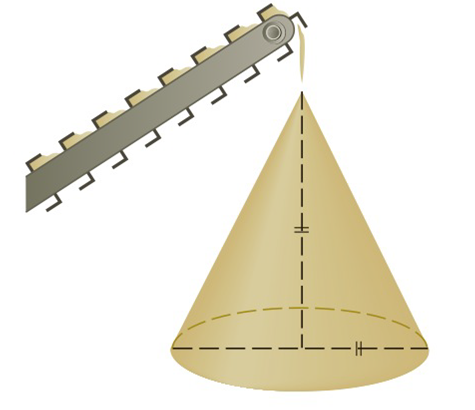Chapter 2.8, Problem 29E

Chapter
Section
Textbook Problem

# Gravel is being dumped from a conveyor belt at a rate of 30 f t 3 / min , and its coarseness is such that it forms a pile in the shape of a cone whose base diameter and height are always equal. How fast is the height of the pile increasing when the pile is 10 ft high?To determine

To find:

The rate at which height of pile is increasing.

Explanation

1. Concept:

We can express the radius in terms of height and then replace this value in the formula of volume of cone. Then differentiate with respect to time and find the required rate.

2. Formula:

i. Volume of cone,V=13πr2h

ii. Power rule of differentiation:

ddxxn=n*xn-1

iii. Chain rule of differentiation:

ddtfx=ddxfx*dxdt

3. Given:

dvdt=30ft3/minh=10 ft

4. Calculations:

It is given that diameter and height are always equal

### Still sussing out bartleby?

Check out a sample textbook solution.

See a sample solution

#### The Solution to Your Study Problems

Bartleby provides explanations to thousands of textbook problems written by our experts, many with advanced degrees!

Get Started

#### A sample with a mean of M = 8 has X = 56. How many scores are in the sample?

Essentials of Statistics for The Behavioral Sciences (MindTap Course List)

#### In problems 37-48, compute and simplify so that only positive exponents remain. 45.

Mathematical Applications for the Management, Life, and Social Sciences